Refer to our Texas Go Math Grade 7 Answer Key Pdf to score good marks in the exams. Test yourself by practicing the problems from Texas Go Math Grade 7 Module 11 Quiz Answer Key.

11.1 Analyzing Categorical Data

The circle graph shows the results of 25 student votes for the new class pet. Find the number of votes for each animal.Question 1.
bird _______
Total number of students who voted = 25
Percentage of vote for the bird as pet = 56%
percentage of vote for bird= 0.56 × 25
= 14
Hence, number of votes for bird is 14

Question 2.
hamster ______
Total number of students who voted = 25
Percentage of vote for the hamster as pet = 12%= 0.12 × 25
= 3
Hence, number of votes for hamster is 3

Question 3.
ferret _______
Total number of students who voted = 25
Percentage of vote for the ferret as pet = 8%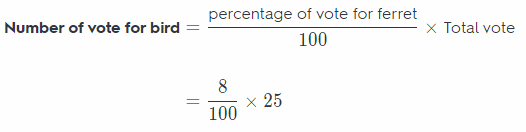= 0.08 × 25
= 2
Hence, number of votes for ferret is 2

Question 4.
snake ______
Total number of students who voted = 25
Percentage of vote for the snake as pet = 24%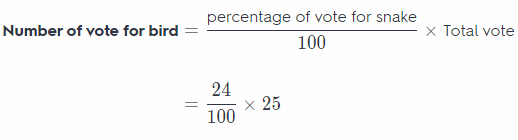= 0.24 × 25
= 6
Hence, number of votes for snake is 6

11.2 Comparing Data Displayed in Dot Plots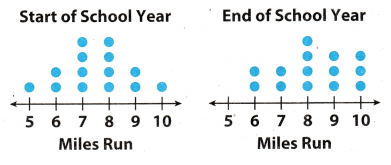The two dot plots show the number of miles run by 14 students at the beginning and at the end of the school year. Compare each measure of the two dot plots.

Question 5.
means ______
Arranging the data of mites run by all the 14 students:
Miles run at start of school year : 5, 6, 6, 7, 7, 7, 7, 8, 8, 8, 8, 9, 9, 10
Miles run at end of school year : 6, 6, 7, 7, 8, 8, 8, 8, 9, 9, 9, 10, 10, 10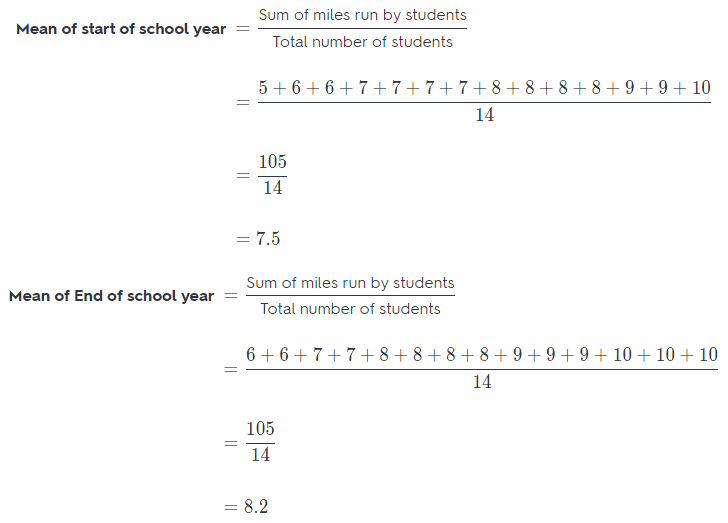Question 6.
medians __________
Arranging the data of miles run by all the 14 students:
Mites run at start of school year: 5, 6, 6, 7, 7, 7, 7, 8, 8, 8, 8, 9, 9, 10
Miles run at end of school year: 6, 6, 7, 7, 8, 8, 8, 8, 9, 9, 9, 10, 10, 10
Here, total number of terms are 14 which is an even number so the medians of the data will be the average of 7th and 8th term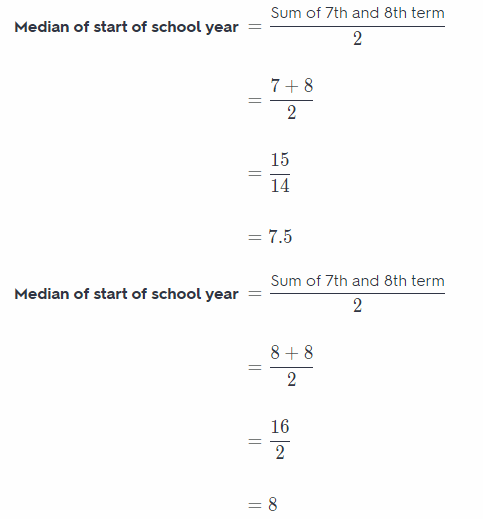Question 7.
ranges ________
Arranging the data of miles run by att the 14 students:
Mites run at start of school year: 5, 6, 6, 7, 7, 7, 7, 8, 8, 8, 8, 9, 9, 10
Miles run at end of school year: 6, 6, 7, 7, 8, 8, 8, 8, 9, 9, 9, 10, 10, 10
Here, the range of mites run by the students will be the difference of maximum and minimum miles run by the students.
Range of start of school year = Max value – Min value
= 10 – 5
= 5
Range of start of school year = Max value – Min value
= 10 – 6
= 4

11.3 Comparing Data Displayed in Box Plots

Use the box plots of inches flown by two different model airplanes for the following exercises.Question 8.
Which has a greater median flight length? _____________
On observating the given box plot the length of air plane are:
Airplane A: 200, 208, 210, 225, & 234.
Airplane B: 180, 195, 204, 230, & 235.
Here total number of term is 5 which is an odd number, we know that when total number of terms is an odd then the median of the given data is middle term. So the median of length of airplane will be the 3rd term
Median of airplane A = 3rd term = 210
Median of airplane B = 3rd term = 204
Hence, the median length of airplane A is greater as compared to the length of airplane B.

Question 9.
Which has a greater interquartile range? ______
On observating the given box plot the length of air plane are:
Airplane A: 200, 208, 210, 225, & 234.
Airplane B: 180, 195, 204, 230, & 235.
Here the box shows the interquartile range. The interquartile angle is the difference between the upper and Lower quartile, which is represented by the length of the box.
Interquartile range of airplane A = Q3 – Q1 = 225 – 208 = 17
Interquartile range of airplane B = Q3 – Q1 = 230 – 195 = 35
Hence, interquartile range of airplane B is greater then airplane A

Question 1o.
Which appears to have a more predictable flight length? ______
The more predictable flight length is by airplane A because the spread of airplane A is less as compared to the
airplane B Also the interquartile angle of airplane A is less as compared to the airplane B So when the length of
flight is compared airplane A is more predictable then airplane B

Essential Question

Question 11.
How can you use and compare data to solve real-world problems?
In the real world, there are so many choices for you to decide which is better or not. Using and comparing data of
the choices helps to make a better decision. You can compare the data statistically to determine which is better.
This is a great help in decision making. For example, when you want to buy a car, you would gather information
about different cars and will compare their data to help you decide which should you purchase. Comparing data
will save you from making a wrong decision.

Using and comparing data helps to make a better decision.

Texas Go Math Grade 7 Module 11 Mixed Review Texas Test Prep Answer Key

Selected Response
Chelsea is reading a 250-page book that is divided into five chapters. For 1-3, use the bar graph below.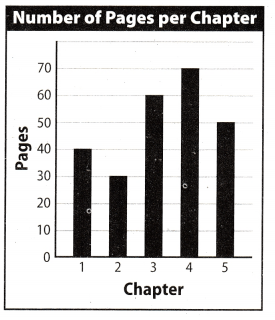Question 1.
What percent of the book’s pages are in Chapter 4?
(A) 24%
(B) 28%
(C) 35%
(D) 70%
(B) 28%

Explanation:
Total number of pages given in book = 250
Number of pages in chapter 4 of book = 70Hence, Option B (28%) is correct option

Question 2.
What percent of the book’s pages are in Chapters 3 and 4?
(A) 24%
(B) 28%
(C) 52%
(D) 65%
(C) 52%

Explanation:
Total number of pages given in book = 250
Number of pages in chapter 3 of book = 60
Number of pages in chapter 4 of book = 70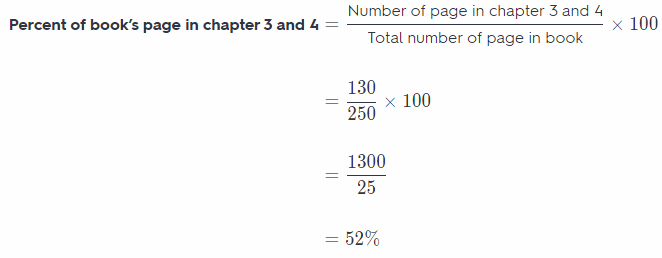Hence, Option C (52%) is correct option

Question 3.
If Chelsea has read through the first half of Chapter 3, what percent of the book has she read?
(A) 25%
(B) 40%
(C) 44%
(D) 52%
(B) 40%

Explanation:
Total number of pages given in book = 250
Number of pages in chapter 1 of book = 40
Number of pages in chapter 2 of book = 30
Number of pages in chapter 3 of book = 60
Total number of pages till 1st half of chapter 3 = 100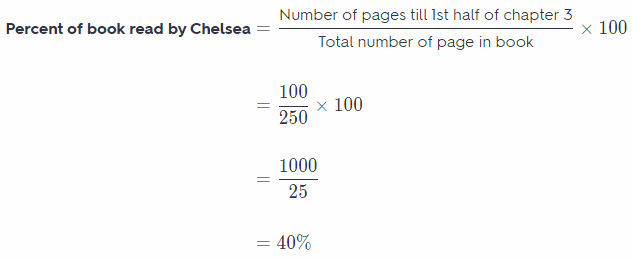Hence, Option B (40%) is correct option

Question 4.
What is -3$$\frac{1}{2}$$ written as a decimal?
(A) -3.05
(B) -3.5
(C) -0.35
(D) -0.035
(B) -3.5

Explanation:Hence, option B(-3.5) is correct option

Question 5.
Which is a true statement based on the dot plots below?(A) Set A has the lesser range.
(B) Set B has the greater median.
(C) Set A has the greater mean.
(D) Set B is less symmetric than Set A
(B) Set B has the greater median.

Explanation:
On comparing both set A and set B we have concLusion:
Option A: is wrong because range of set A is 10-60 and set B is 20-60
Option B : is correct because median of set B is greater then set A
Option C: is wrong because mean of set A is greater then set B
Option D: is wrong because set B is more symmetric at 40
Hence, option B is correct option

Gridded Response

Question 6.
The dot plot shows the number of pencils each boy has at his desk in class.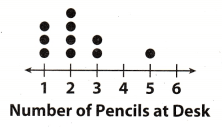Find the median for the number of pencils.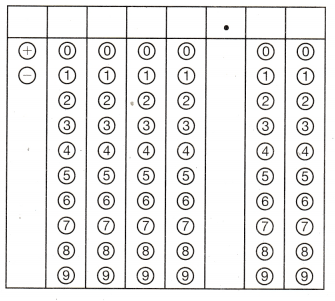From the given dot plot we can see that 3 boys has 1 pencils on desk, 4 boy has 2 pencils on desk, 2 boy has 3
pencils on desk and at last boy has 5 pencils on his desk. So arranging the given data in order.
1, 1, 1, 2, 2, 2, 2, 3, 3, 5
Total numbers of term are 10, so median will be average of 5th and 6th term.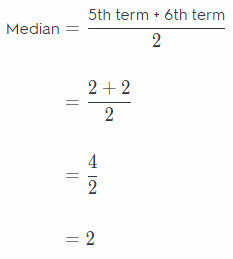Hence, median for number of pencils is 2
Table will be made as per below instruction:
1st column: mark + sign
2nd column : mark 0
3rd column : mark 0
4th column : mark 0
5th column : mark 2
6th column : mark 0
7th column : mark 0

Scroll to Top